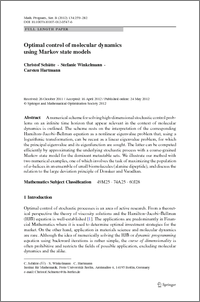Repository: Freie Universität Berlin, Math Department

# Optimal control of molecular dynamics using Markov state models

Schütte, Ch. and Winkelmann, S. and Hartmann, C. (2012) Optimal control of molecular dynamics using Markov state models. Math. Program. (Series B), 134 (1). pp. 259-282.Preview
PDF - Published Version
681kB

Official URL: http://dx.doi.org/10.1007/s10107-012-0547-6

## Abstract

A numerical scheme for solving high-dimensional stochastic control problems on an infinite time horizon that appear relevant in the context of molecular dynamics is outlined. The scheme rests on the interpretation of the corresponding Hamilton-Jacobi-Bellman equation as a nonlinear eigenvalue problem that, using a logarithmic transformation, can be recast as a linear eigenvalue problem, for which the principal eigenvalue and its eigenfunction are sought. The latter can be computed efficiently by approximating the underlying stochastic process with a coarse-grained Markov state model for the dominant metastable sets. We illustrate our method with two numerical examples, one of which involves the task of maximizing the population of $\alpha$-helices in an ensemble of small biomolecules (Alanine dipeptide), and discuss the relation to the large deviation principle of Donsker and Varadhan.

Item Type: Article Mathematical and Computer Sciences > Mathematics > Applied Mathematics Other Institutes > Matheon > A - Life SciencesDepartment of Mathematics and Computer Science > Institute of Mathematics > Cellular Mechanics GroupDepartment of Mathematics and Computer Science > Institute of Mathematics > BioComputing Group 1107 Carsten Hartmann 26 Oct 2011 12:51 03 Mar 2017 14:41

### Available Versions of this Item

• Optimal control of molecular dynamics using Markov state models. (deposited 26 Oct 2011 12:51) [Currently Displayed]

Repository Staff Only: item control page